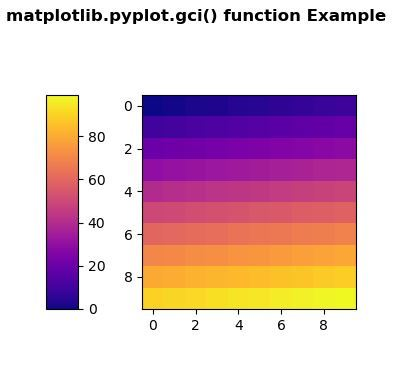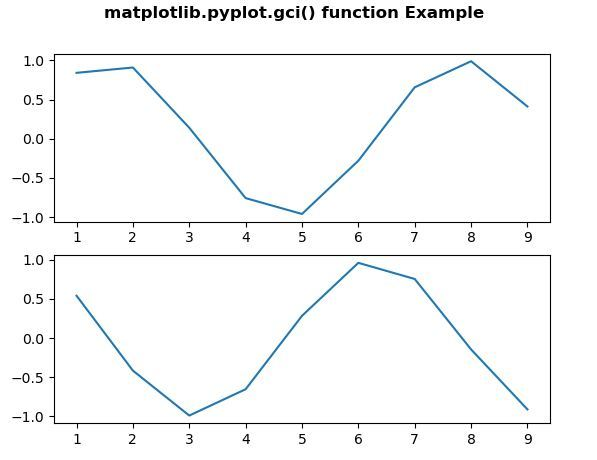Related Articles

# Matplotlib.pyplot.gci() in Python

• Last Updated : 10 May, 2020

Matplotlib is a library in Python and it is numerical – mathematical extension for NumPy library. Pyplot is a state-based interface to a Matplotlib module which provides a MATLAB-like interface. There are various plots which can be used in Pyplot are Line Plot, Contour, Histogram, Scatter, 3D Plot, etc.

## matplotlib.pyplot.gci() method

Attention geek! Strengthen your foundations with the Python Programming Foundation Course and learn the basics.

To begin with, your interview preparations Enhance your Data Structures concepts with the Python DS Course. And to begin with your Machine Learning Journey, join the Machine Learning - Basic Level Course

The gci() method in pyplot module of matplotlib library is used to get the current colorable artist.

Syntax: matplotlib.pyplot.gci()

Parameters: This method does not accept any parameters.

Returns: This method returns the current colorable artist.

Below examples illustrate the matplotlib.pyplot.gci() function in matplotlib.pyplot:

Example 1:

 `# Implementation of matplotlib function ``import` `matplotlib.pyplot as plt ``import` `numpy as np ``import` `matplotlib.gridspec as gridspec ``from` `mpl_toolkits.axes_grid1 ``import` `make_axes_locatable ``   ` `   ` `plt.close(``'all'``) ``arr ``=` `np.arange(``100``).reshape((``10``, ``10``)) ``fig ``=` `plt.figure(figsize ``=``(``4``, ``4``)) ``im ``=` `plt.imshow(arr,  ``                ``interpolation ``=``"none"``, ``                ``cmap ``=``"plasma"``) ``   ` `divider ``=` `make_axes_locatable(plt.gca()) ``cax ``=` `divider.append_axes(``"left"``,  ``                          ``"15 %"``, ``                          ``pad ``=``"30 %"``) ``   ` `plt.colorbar(im, cax ``=` `cax) `` ` `print``(``"The current colorable artist is :"``)``print``(plt.gci())`` ` `fig.suptitle('matplotlib.pyplot.gci() function \``Example\n\n', fontweight ``=``"bold"``) `` ` `plt.show() `

Output:```The current colorable artist is :
AxesImage(50, 44;310x308)
```

Example 2:

 `# Implementation of matplotlib function``import` `matplotlib.pyplot as plt``from` `scipy ``import` `sin, cos`` ` ` ` `fig, ax ``=` `plt.subplots(``2``, ``1``)``x ``=` `[``1``, ``2``, ``3``, ``4``, ``5``, ``6``, ``7``, ``8``, ``9``]`` ` `y1 ``=` `sin(x)``y2 ``=` `cos(x)`` ` `plt.sca(ax[``0``])``plt.plot(x, y1)`` ` `plt.sca(ax[``1``])``plt.plot(x, y2)`` ` `print``(``"The current colorable artist is :"``)``print``(plt.gci())`` ` `fig.suptitle('matplotlib.pyplot.gci() function \``Example\n\n', fontweight ``=``"bold"``) `` ` `plt.show() `

Output:```The current colorable artist is :
None
```

My Personal Notes arrow_drop_up